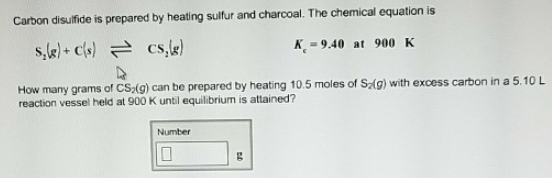# Problem: Carbon disulfide is prepared by heating sulfur and charcoal. The chemical equation is S2(g) + C(s) ⇌ CS2(g)          Kc = 9.40 at 900 K How many grams of CS2(g) can be prepared by heating 10.5 moles of S2(g) with excess carbon in a 5.10 L reaction vessel held at 900 K until equilibrium is attained?

###### FREE Expert Solution
97% (223 ratings)###### Problem Details

Carbon disulfide is prepared by heating sulfur and charcoal. The chemical equation is

S2(g) + C(s) ⇌ CS2(g)          Kc = 9.40 at 900 K

How many grams of CS2(g) can be prepared by heating 10.5 moles of S2(g) with excess carbon in a 5.10 L reaction vessel held at 900 K until equilibrium is attained?